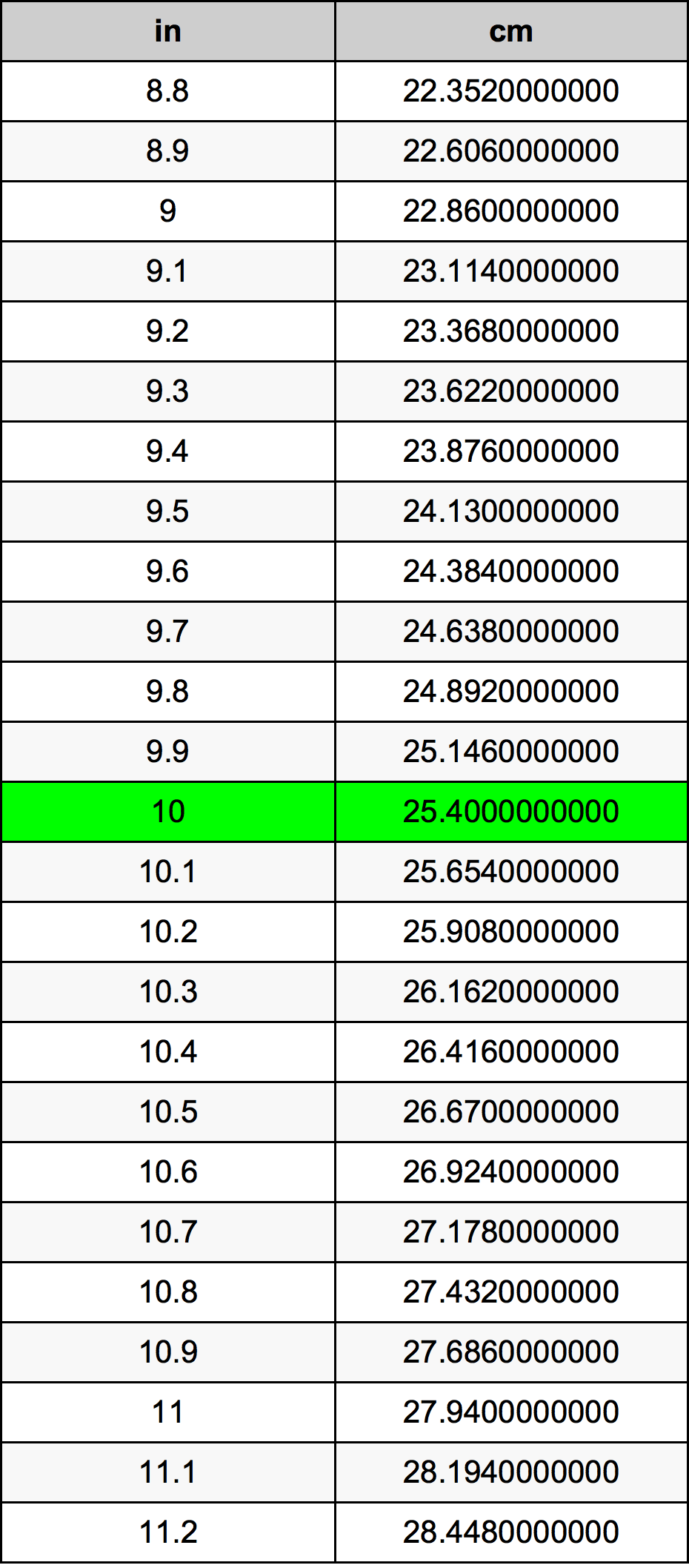Inches To Centimeters

# 10 in to cm10 Inches to Centimeters

in
=
cm

## How to convert 10 inches to centimeters?

 10 in * 2.54 cm = 25.4 cm 1 in
A common question is How many inch in 10 centimeter? And the answer is 3.937007874 in in 10 cm. Likewise the question how many centimeter in 10 inch has the answer of 25.4 cm in 10 in.

## How much are 10 inches in centimeters?

10 inches equal 25.4 centimeters (10in = 25.4cm). Converting 10 in to cm is easy. Simply use our calculator above, or apply the formula to change the length 10 in to cm.

## Convert 10 in to common lengths

UnitLengths
Nanometer254000000.0 nm
Micrometer254000.0 µm
Millimeter254.0 mm
Centimeter25.4 cm
Inch10.0 in
Foot0.8333333333 ft
Yard0.2777777778 yd
Meter0.254 m
Kilometer0.000254 km
Mile0.0001578283 mi
Nautical mile0.000137149 nmi

## What is 10 inches in cm?

To convert 10 in to cm multiply the length in inches by 2.54. The 10 in in cm formula is [cm] = 10 * 2.54. Thus, for 10 inches in centimeter we get 25.4 cm.

## 10 Inch Conversion Table## Alternative spelling

10 Inches to cm, 10 Inches in cm, 10 Inch to Centimeters, 10 Inch in Centimeters, 10 Inch to cm, 10 Inch in cm, 10 in to Centimeters, 10 in in Centimeters, 10 in to Centimeter, 10 in in Centimeter, 10 Inch to Centimeter, 10 Inch in Centimeter, 10 in to cm, 10 in in cm